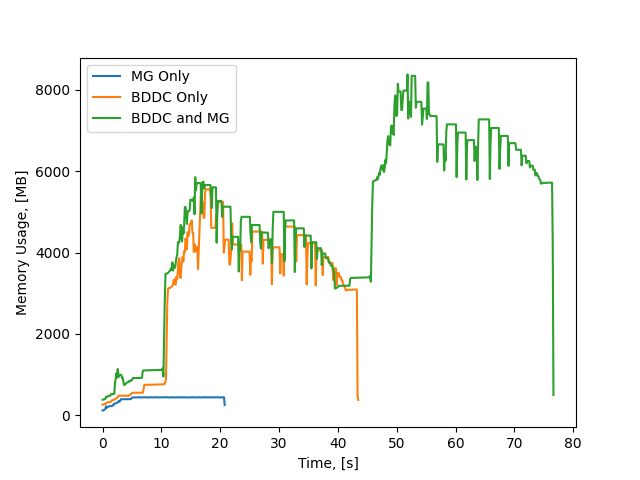# Using BDDC in combination with Multigrid

Hi All,

I’m currently considering eddy current type electomagnetic problems for highly conducting magnetic objects where we have thin skin depths normal to the object surface. We’ve accounted for these thin skin via layers of prismatic boundary layer elements with high order HCurl conforming elements, which works well but leads to dense meshes and a high memory usage.

Up to this point, we have been using the BDDC preconditioner, which was sufficient for simpler problems, but we’ve been running into the memory limits of our hardware. My understanding is that the BDDC preconditioner solves for the lowest order degrees of freedom directly, which for dense meshes would be expensive. From what I’ve read in Tutorial 2.1.4, we can reduce this cost by swaping out the direct solve for a (geometric) multigrid implementation by setting ‘coarsetype="multigrid’ as an argument for the preconditioner. e.g.

c = Preconditioner(a, 'bddc', coarsetype='multigrid')

and generating a sequence of meshes via the Refine method. Is this correct?

As an example, I’ve attached some sample code, where I’m solving a simple curl curl problem: Find \mathbf{A} s.t.

\nabla \times \nabla \times \mathbf{A} + \mathbf{A} = \mathbf{f} \text{ in } \Omega
\boldsymbol{n}\times \mathbf{A} = \boldsymbol{n}\times \mathbf{A}_0 \text{ on } \partial \Omega

where \mathbf{A}_0 = [sin(y), 0, 0] is the exact solution and \mathbf{f} is a known source term over a unit cube \Omega. My example code is adapted from the function provided in Tutorial 2.1.1 and iterates through a series of refined meshes. In the code I’m comparing the number of iterations required to solve the problem using the CGSolver and either the BDDC, Multigrid, or the combined preconditioners. What I see is that the combined preconditioner takes significantly more iterations.

Futhermore, I’ve reran this test for the case of p=0. For p=0 the BDDC preconditioner should solve the problem directly on each mesh, and the multigrid and combined preconditioners should be equivalent to each other. What I see is that the combined preconditioner takes substantially more iterations. Have I made some mistake here, either with my implementation or my understanding?

Regards,
James
bddc_mult_example.py (2.0 KB)

Just to add on to my original post, I’ve tried implementing my own multigrid preconditioner, adapted from this forum post.

In addition to keeping track of the number of iterations, I’ve also been looking at the memory usage. I’ve included a figure showing the memory usage as a function of time for a similar problem to my original post using order 0. What I see is that there is a large increase in memory usage when combining the BDDC and multigrid preconditioners.I accept that it’s difficult to compare the memory usage and speed between something written in python and something written in C, but given that my multigrid implementation has a near constant memory usage, I don’t understand what the second hump corresponds to in the combined preconditioner.

James

The elementwise bddc implemented in ngsolve eliminates high order dofs and reduces it to lowest order. The lowest order is then solved directly. For p=0 the ngsolve bddc is just a direct solver.
Also the multigrid already does only smoothing for the high order dofs and does geometric mg prolongation only on lowest order dofs. So combining these two ngsolve-preconditioners does no really make sense. Does this answer your questions?

Hi Christopher,